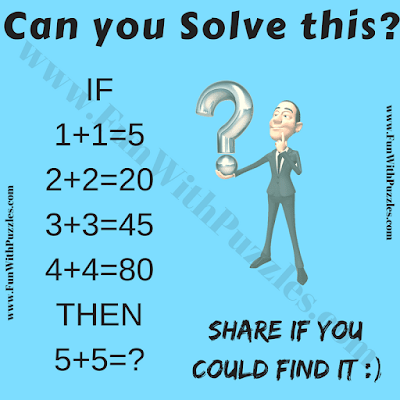It is Brain Math Puzzle for school-going Teens. This Brain Math Number Question Game Puzzle will test your Logical IQ. In this Brain Math Puzzle, your challenge is to decipher the hidden logical sequence in the given equations. After finding this logical sequence, solve the last equation and find the value of the missing number. Can you solve this Digits Puzzle?Can you solve this Digit Puzzle for Teens?

The answer to this "Brain Math Puzzle for Teens", can be viewed by clicking the button. Please do give your best try before looking at the answer.

#### 1 comment:

Unknown said...

It is so easy to do